# ISEVEN Function Examples – Excel, VBA, & Google Sheets

This tutorial demonstrates how to use the ISEVEN and ISODD Functions to test if numerical values are odd or even in Excel and Google Sheets.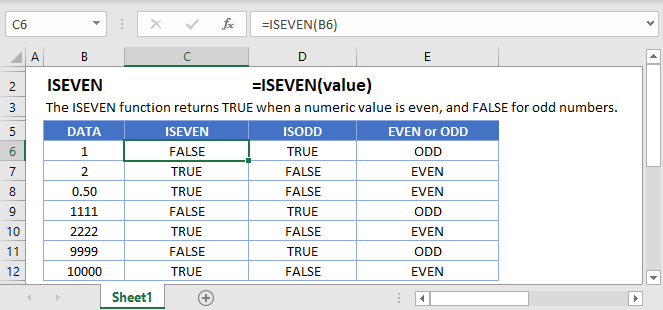## ISEVEN Function Overview

The ISEVEN Function Test if cell value is even. Returns TRUE or FALSE.

To use the ISEVEN Excel Worksheet Function, select a cell and type: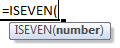(Notice how the formula inputs appear)

### ISEVEN Function Syntax and Inputs:

value – The test value

## How to use the ISEVEN and ISODD Functions

The ISEVEN Function tests if a numerical value is even. If it’s even, the function returns TRUE, otherwise it returns FALSE.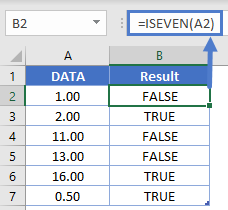The ISODD Function does the opposite.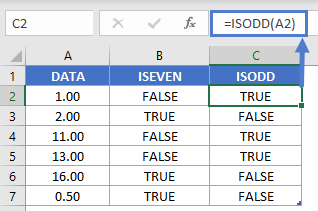The most common uses of the ISEVEN and ISODD Functions are to shade alternate rows using Conditional Formatting.

To do this, you will use the formula:

=ISEVEN(ROW()))

within Conditional Formatting:

1. Select all the cells to shade
2. Go to Home Ribbon > Conditional Formatting > New Rule
3. Select ‘Use a formula to determine which cells to format
4. Enter the formula =ISEVEN(ROW())
5. Click the Format button and choose your desired formatting
6. Click OK twice to quit and save.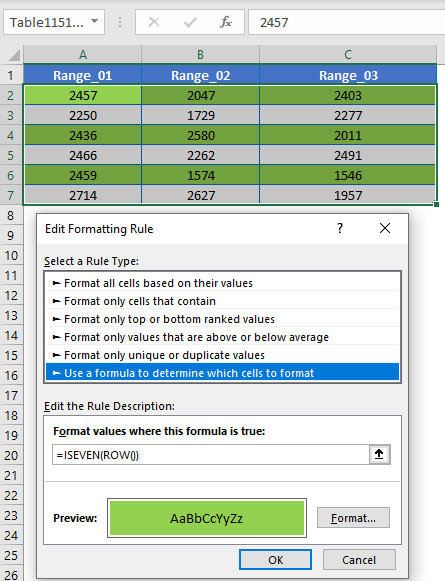You can add a second conditional formatting rule to also shade the ODD Rows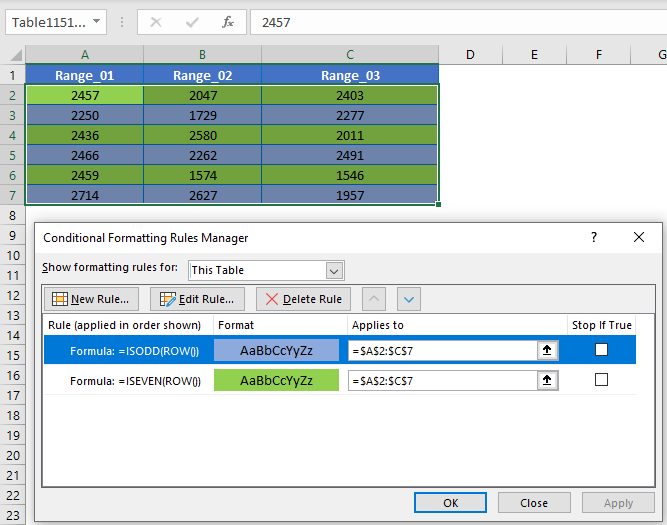The ISEVEN Function works exactly the same in Google Sheets as in Excel: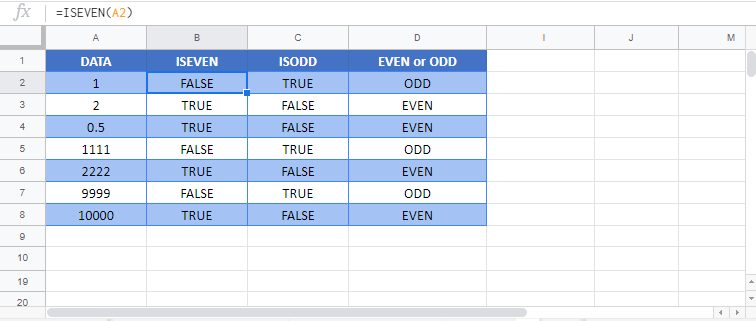## ISEVEN Examples in VBAYou can also use the ISEVEN function in VBA. Type:
`Application.Worksheetfunction.Iseven(number)`
For the function argument (number), you can either enter them directly into the function, or define variables to use instead.